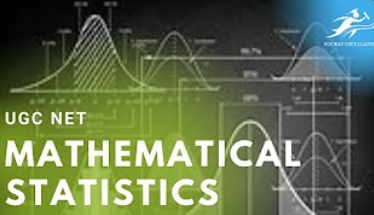top of page

# UGC NET MATHEMATICAL STATISTICS online and offline special sessionsStatistical science is concerned with the planning of studies, especially with the design of randomized experiments and with the planning of surveys using random sampling. The initial analysis of the data from properly randomized studies often follows the study protocol.

The data from a randomized study can be analyzed to consider secondary hypotheses or to suggest new ideas. A secondary analysis of the data from a planned study uses tools from data analysis. Data analysis is divided into: descriptive statistics - the part of statistics that describes data, i.e. summarises the data and their typical properties. inferential statistics - the part of statistics that draws conclusions from data (using some model for the data):

For example, inferential statistics involves selecting a model for the data, checking whether the data fulfill the conditions of a particular model, and with quantifying the involved uncertainty (e.g. using confidence intervals). While the tools of data analysis work best on data from randomized studies, they are also applied to other kinds of data --- for example, from natural experiments and observational studies, in which case the inference is dependent on the model chosen by the statistician, and so subjective Mathematical statistics has been inspired by and has extended many options in applied statistics.

## UGC NET 2014 JUNE PAPER 2 QUESTIONS 45TO50 Statistical economics

UGC CSIR NET ,IIT ,NPTEL MATHEMATICAL SCIENCE STATISTICAL INTERFERENCE & GAUSS MARKOV MODEL CBSE NET

## UGC CSIR NET ,IIT ,NPTEL MATHEMATICAL SCIENCE STATISTICAL INTERFERENCE & GAUSS MARKOV MODEL CBSE NET

Did you like our post?

• Yes, I liked it

• yes, I really liked it

0 views